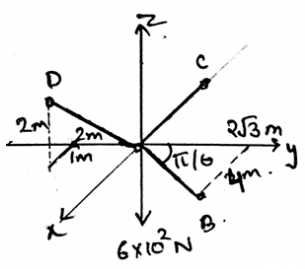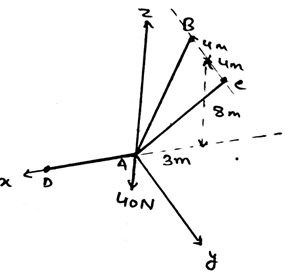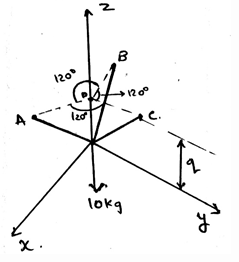Courses

# Test: Equilibrium In Three Dimensions

## 15 Questions MCQ Test Engineering Mechanics | Test: Equilibrium In Three Dimensions

Description
This mock test of Test: Equilibrium In Three Dimensions for Mechanical Engineering helps you for every Mechanical Engineering entrance exam. This contains 15 Multiple Choice Questions for Mechanical Engineering Test: Equilibrium In Three Dimensions (mcq) to study with solutions a complete question bank. The solved questions answers in this Test: Equilibrium In Three Dimensions quiz give you a good mix of easy questions and tough questions. Mechanical Engineering students definitely take this Test: Equilibrium In Three Dimensions exercise for a better result in the exam. You can find other Test: Equilibrium In Three Dimensions extra questions, long questions & short questions for Mechanical Engineering on EduRev as well by searching above.
QUESTION: 1

### A force is developed by a support that not allows the ________ of its attached member.

Solution:

The force developed by a support doesn’t allow the translation of its attached member. This is the basic condition for the equilibrium of the forces in any dimension. This rule is applied when the support reactions are taken into the account for the equilibrium of the body.

QUESTION: 2

### In the diagram given below, coordinates of D is (1, -2, 2), C (-2, 0, 0) and B are as shown. The dark region is the cables holding the weight of 600N at origin. Find the tension in the AD section.Solution:

As the system is in equilibrium so we need to balance the forces. So when apply the condition of net force to be zero in the z direction, we get (2/3)FAD = 600N. This gives us force along AD be 900N.

QUESTION: 3

### A couple moment is developed when _______ of the attached member is prevented.

Solution:

The development of the couple moment is when there is prevention of the rotation of the attached member. This is the basic condition for the equilibrium of the couple moments in any dimension. This rule is applied when the couple moments are taken into the account for the equilibrium of the body.

QUESTION: 4

What is not the condition for the equilibrium in three dimensional system of axis?

Solution:

For the equilibrium in the three dimensional system of axis we have all the conditions true as, ∑Fx=0, ∑Fy=0 and ∑Fz=0. Also we have the summation of the forces equal to zero. Which is not a non-zero value.

QUESTION: 5

We first make equilibrium equations of the body by considering all the three dimensional forces and then the free body diagram is made and solved.

Solution:

We first make the free body diagram and then we make the equilibrium equations to satisfy the given conditions. This helps us to solve the question easily. As this reduces the part of imagination and increases accuracy too.

QUESTION: 6

If solving the question in 3D calculations is difficult, then use the 2D system and then equate the total net force to zero.

Solution:

The answer is obviously yes. If we are having any difficulty in making the vector components, then we can go in 2D. As if the particle is in equilibrium, the net force will be zero. No matter where you see first. Net force is zero.

QUESTION: 7

∑Fx=0, ∑Fy=0 and ∑Fz=0 are vector equations for the three dimensions. They are satisfied when the body is achieved it state of equilibrium.

Solution:

The answer is false as the equations asked are scalars. As we make the net sum of the forces along the axis equal to zero. Of course this equation comes from the solving the vector forms, but still the result is a scalar, hence the equations are scalar.

QUESTION: 8

If the resolved force or the force which you get as the answer after solving the question is negative, then what does this implies?

Solution:

The negative sign implies things in the opposite manner. If the force is coming negative this doesn’t means that it is impossible. But it means that the force is in the opposite direction w.r.t the direction set by you in the free body diagram.

QUESTION: 9

The supports in the 3D are having more than three reaction forces. Because they are having three axis on which the components of the forces need to be zero.

Solution:

As 3D is defined as the three axis system, we have to consider the equilibrium in all the three axis. This will make the equilibrium go on all the axis of the 3D space. And hence will cancel all the forces.

QUESTION: 10

If any body is tied to three or more ropes, and then is allowed to achieve its equilibrium. Then the equilibrium achieved is achieved w.r.t what?

Solution:

Yes, the equilibrium is being achieved w.r.t the ground. Like the motion w.r.t ground need be zero. That is the relative velocity of the object or the body must be zero w.r.t the ground. This means motion is in equilibrium.

QUESTION: 11

The single pin, single bearing and single hinge resist _______

Solution:

The experiments have founded that the single pin, single bearing and single hinge resist both force and couple moments. This does helps in attaining the equilibrium of the body. Thus proper guiding must be taken during solving the question on the same.

QUESTION: 12

If the supports are properly aligned then the reaction forces developed are adequate to support the body.

Solution:

This is the basic nature observed during the experiments on the beams an all the support system. The dimension doesn’t affect the equilibrium conditions. The main motto is to achieve the equilibrium. It is achieved by equating the net force equal to zero.

QUESTION: 13

Find the tension in the cable AC.Solution:

First represent the forces in their vector form. Then equate the net sum of the forces in the x, y and z directions to be zero. You will get FB = FC and 2(.848) = 40N. This gives the answer as 23.6N.

QUESTION: 14

Determine the value of the q, parallel to the z axis. That is the point of intersection of the projections of the points A, B and C parallel to the xy plane. With the distance between the tri-section point and the points A, B and C be equal to 0.6m.Solution:

The application of the equilibrium equation will yield the result. That is the resultant along the z-axis will remain zero. Which give the value of γ as 50˚. And therefore q=51.9cm.

QUESTION: 15

If the supports are not properly aligned then the reaction forces developed are adequate to support the body.

Solution:

This is the basic nature observed during the experiments on the beams an all the support system. The dimension doesn’t affect the equilibrium conditions. The main motto is to achieve the equilibrium. It is achieved by equating the net force equal to zero. But here the system is not properly aligned.